Test: Diode Rectifiers

# Test: Diode Rectifiers

Test Description

## 10 Questions MCQ Test Power Electronics | Test: Diode Rectifiers

Test: Diode Rectifiers for Electrical Engineering (EE) 2023 is part of Power Electronics preparation. The Test: Diode Rectifiers questions and answers have been prepared according to the Electrical Engineering (EE) exam syllabus.The Test: Diode Rectifiers MCQs are made for Electrical Engineering (EE) 2023 Exam. Find important definitions, questions, notes, meanings, examples, exercises, MCQs and online tests for Test: Diode Rectifiers below.
Solutions of Test: Diode Rectifiers questions in English are available as part of our Power Electronics for Electrical Engineering (EE) & Test: Diode Rectifiers solutions in Hindi for Power Electronics course. Download more important topics, notes, lectures and mock test series for Electrical Engineering (EE) Exam by signing up for free. Attempt Test: Diode Rectifiers | 10 questions in 30 minutes | Mock test for Electrical Engineering (EE) preparation | Free important questions MCQ to study Power Electronics for Electrical Engineering (EE) Exam | Download free PDF with solutions
 1 Crore+ students have signed up on EduRev. Have you?
Test: Diode Rectifiers - Question 1

### A diode rectifier cannot perform rectification in both directions' is a perfect example of:

Detailed Solution for Test: Diode Rectifiers - Question 1

Unilateral Circuit:

• In unilateral circuits, the property of the circuit changes with the change of direction of supply voltage or current.
• In other words, a unilateral circuit allows the current to flow only in one direction.
• A diode rectifier is the best example of a unilateral circuit because it does not perform the rectification in both directions of supply.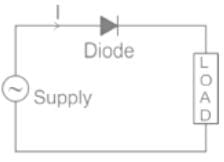• The circuit consisting of Diodes like the rectifier circuit is an example of a Unilateral Circuit.
• In this circuit, the current can flow in one direction only because the diode allows the flow of current in one direction only.
Test: Diode Rectifiers - Question 2

### In comparing the operation of the half-controlled 2-pulse circuit with that of the fully-controlled circuit, which of the following statements are evident? 1. Since half the thyristors are replaced by diodes, a half-controlled converter costs less than a fully-controlled converter. 2. Due to the freewheeling action with a half-controlled bridge-circuit power factor is improved in half-controlled converters. 3. The AC supply current is more distorted due to its zero periods with the half-controlled circuit, compared to a fully-controlled bridge circuit. Select the correct answer using the code given below :

Detailed Solution for Test: Diode Rectifiers - Question 2

Difference Between Half Wave and Full Wave Rectifier: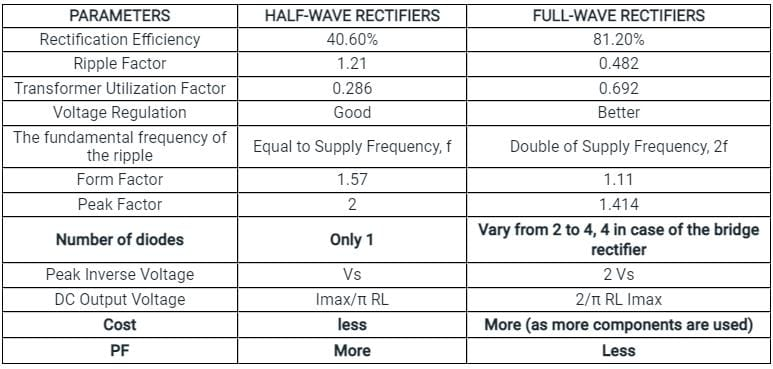Test: Diode Rectifiers - Question 3

### The average current rating of a semiconductor diode will be maximum for:

Detailed Solution for Test: Diode Rectifiers - Question 3

Maximum Average Forward Current (IFAVM):
Diodes are often used in rectifier circuits supplying a DC (average) current to the load.
In such cases, the average load current and the diode forward current usually have a simple relationship.
Therefore, it will be of interest to know the maximum average current, a diode can conduct in the forward direction.
This specification gives the maximum average value of power frequency half cycle sine wave current allowed to flow through the diode in the forward direction.
The average current rating of a diode decreases with a reduction in conduction angle due to an increase in the current “form factor”.

Full-wave rectifier output waveform: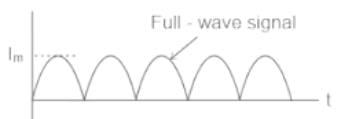Average current value = 2Im/π
Half-wave rectifier output waveform: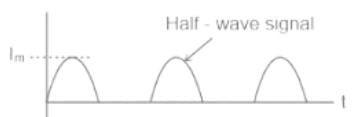Average current value = Im/π

DC output waveform: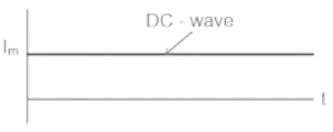Average current value = Im
So average current rating will be in the order of DC > Full-wave rectifier > Half-wave rectifier.

Test: Diode Rectifiers - Question 4

The ripple factor of a power supply is the ratio of:

Detailed Solution for Test: Diode Rectifiers - Question 4

Ripple factor:

The amount of AC present in the output of the signal is called as ripple.

The ripple factor indicates the number of ripples present in the DC output.

The output of the power supply is given by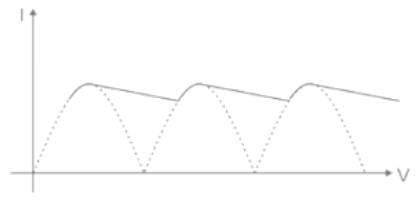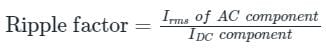It is given as: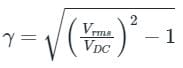Thus if the ripple factor is less, the power supply has less AC components and power supply output is purer (i.e more DC without much fluctuations)

Thus ripple factor is an indication of the purity of output of the power supply.

Test: Diode Rectifiers - Question 5

For a total harmonic distortion of 0.1 with I1 = 4A and Rc = 8Ω, calculate total power

Detailed Solution for Test: Diode Rectifiers - Question 5

Concept:

Total harmonics distortion is given by,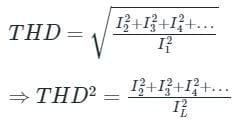Now, total power due to all harmonic component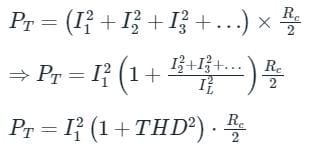Calculation:
Given that, THD = 0.1
IL = 4 A, Rc = 8 Ω
⇒ PT = (4)2(1 + (0.1)2) × 8/2

PT = 64.64 W

Test: Diode Rectifiers - Question 6

Average output voltage of a three-phase full wave diode rectifier is given by:

Detailed Solution for Test: Diode Rectifiers - Question 6

Three-Phase Full Wave Diode Rectifier:

• The full-wave three-phase uncontrolled bridge rectifier circuit uses six diodes, two per phase.
• A 3-phase full-wave rectifier is obtained by using two half-wave rectifier circuits. The advantage here is that the circuit produces a lower ripple output than the previous half-wave 3-phase rectifier as it has a frequency of six times the input AC waveform.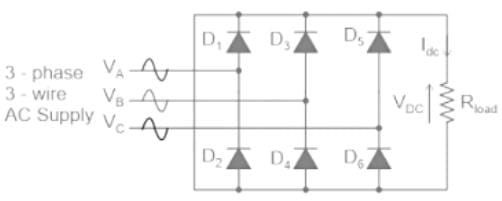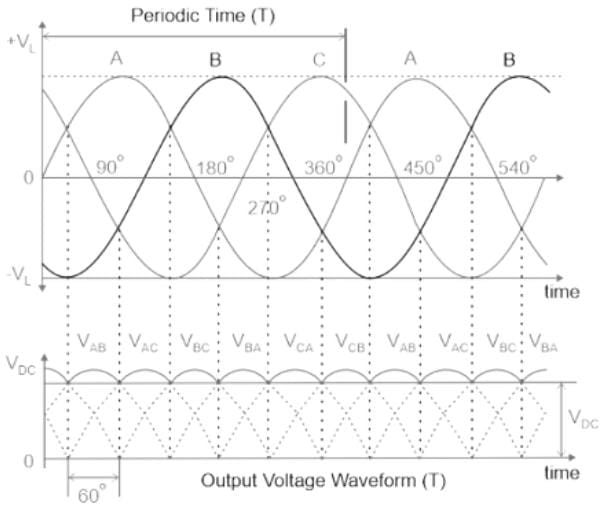• As before, assuming a phase rotation of Red-Yellow-Blue (VA – VB – VC) and the red phase (VA) starts at 0o. Each phase connects between a pair of diodes as shown. One diode of the conducting pair powers the positive (+) side of the load, while the other diode powers the negative (-) side of the load.
• Diodes D1 D3 D2 and D4 form a bridge rectifier network between phases A and B, similarly diodes D3 D5 D4 and D6 between phases B and C and D5 D1 D6, and D2 between phases C and A.
• If we start the pattern of conduction at 30o, this gives us a conduction pattern for the load current of D1-4 D1-6 D3-6 D3-2 D5-2 D5-4, and return again to D1-4 and D1-6 for the next phase sequence as shown.
• So the average DC value of the output voltage waveform from a 3-phase full-wave rectifier is given as: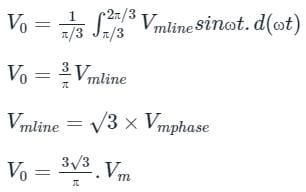Test: Diode Rectifiers - Question 7

Calculate the rectification efficiency of half wave rectifier if input power to rectifier is 150 W and power obtained is 90 W.

Detailed Solution for Test: Diode Rectifiers - Question 7

Formula Used:

efficiency (%η) = Po/Pi

Here, Po is the output power

And, Pi is the input power

Application:

Given,

Input AC Power (Pi) = 150 W

Output DC Power (Po) = 90 W

Hence, Efficiency (η) = 90/150 = 0.6 = 60 %

Test: Diode Rectifiers - Question 8

The transformer Utilization factor of a bridge rectifier is approximately:

Detailed Solution for Test: Diode Rectifiers - Question 8

Transformer Utilization Factor (TUF):

It is defined as the ratio of dc power output to the transformer Volt-Ampere (VA) rating required by the secondary winding.

TUF = (DC power output) / (Effective VA rating of transformer)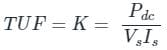It is a quantitative indication of the utilization of the VA Rating of Transformer. The more the value of TUF, the more will be the utilization. In other words, the VA rating of the required transformer will be less if TUF is more and vice versa.

Application:

The output average voltage of a single-phase full-bridge diode rectifier is given by
V0 = 2Vm

Here, The output average current for R load is given by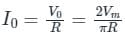Supply voltage Vs RMS is given by Vm/√2

Supply current Is RMS is given by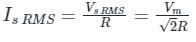Now,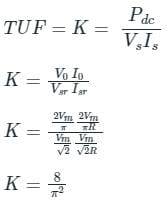= 0.81
= 81%

Test: Diode Rectifiers - Question 9

A single-phase full wave bridge diode rectifier delivers a constant current of 10 A to the load. Average and RMS values of source current are

Detailed Solution for Test: Diode Rectifiers - Question 9

Concept:

In a single-phase uncontrolled diode rectifier

The average output voltage, Vo = 2Vm

Supply RMS current isr = Io

Supply average current = 0

In a three-phase uncontrolled diode rectifier

The average output voltage, Vo = 3Vml

Supply RMS current isr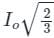Supply average current = 0

Calculation:

Given that, load current (I0) = 10 A

Supply average current = 0 A

Supply RMS current = 10 A

Test: Diode Rectifiers - Question 10

In the process of diode based rectification, the alternating input voltage is converted into

Detailed Solution for Test: Diode Rectifiers - Question 10

Rectification is AC to DC. In DIODE biased rectification, control is not possible.

## Power Electronics

5 videos|39 docs|63 tests
 Use Code STAYHOME200 and get INR 200 additional OFF Use Coupon Code
Information about Test: Diode Rectifiers Page
In this test you can find the Exam questions for Test: Diode Rectifiers solved & explained in the simplest way possible. Besides giving Questions and answers for Test: Diode Rectifiers, EduRev gives you an ample number of Online tests for practice

## Power Electronics

5 videos|39 docs|63 tests